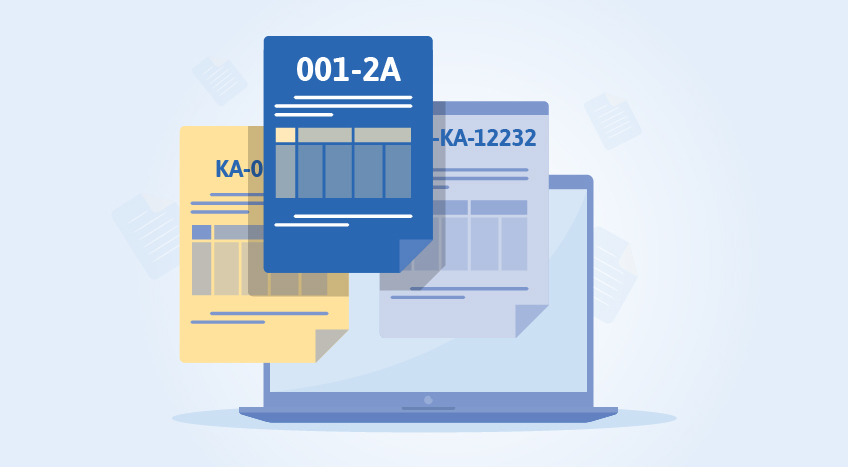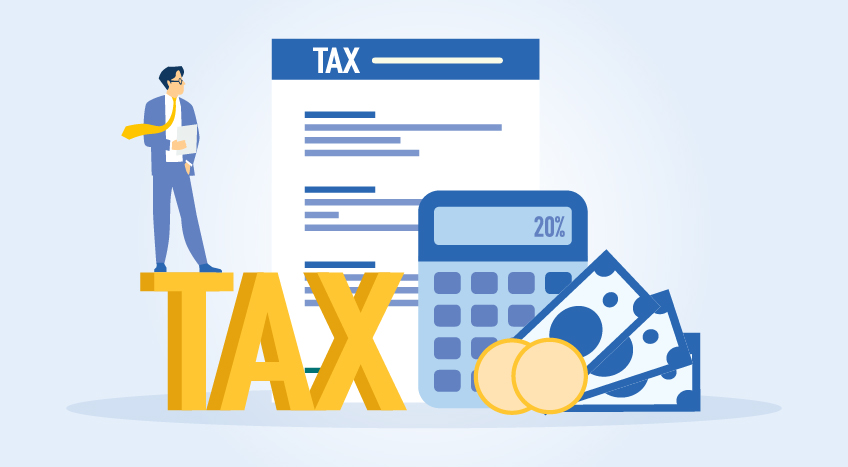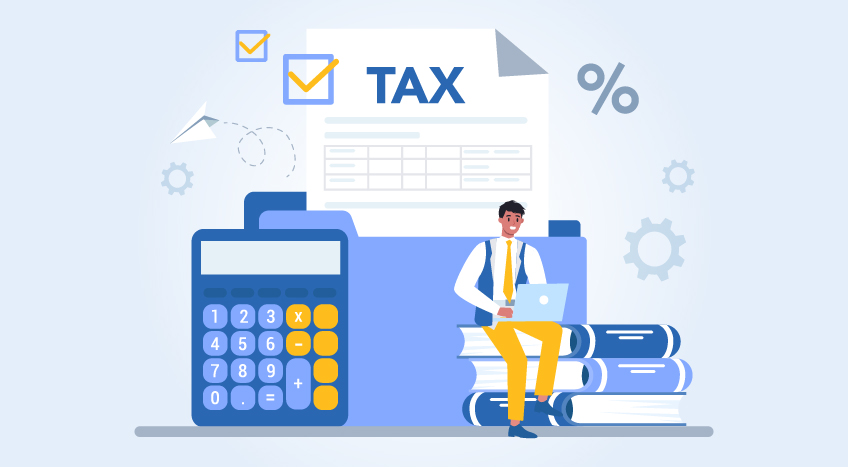# VAT Calculator – How to Calculate VAT

##### Yarab A | Updated on: January 23, 2023

Worried about how much amount to be added as VAT? Got the price inclusive of VAT and unsure of VAT amount?

The online VAT calculator helps you calculate VAT, both exclusive and inclusive of VAT. Just type the value and get your vat calculations.

## How Vat Calculator works

Using the VAT calculator, you can determine the taxable value and the VAT amount. To calculate VAT, all you need to know is the amount i.e. the price and the VAT rate. With the help of the VAT calculator, you can calculate exclusive and inclusive VAT.

### Exclusive of VAT

Here, the amount or price that you enter is excluding VAT i.e.it is a pure cost of the product or service. When you select exclusive of VAT, the VAT rate will be applied using the formula given below.

Taxable Value = Rate of VAT÷100

### Inclusive of VAT

Here, the amount or the price quoted is inclusive of VAT. In other words, it includes the product cost and the VAT amount. When the price quoted is inclusive of VAT, the VAT amount can be derived, using the following formula:

VAT amount = Value inclusive of tax X tax rate ÷ (100 + tax rate)

Taxable Value/Price = Value inclusive of tax X 100 ÷ (100 + tax rate)

## What is input Vat and output VAT?

The fundamental of VAT is largely based on the concept of the input VAT and output VAT. Let’s understand it with examples.

Output VAT

The tax collected on the sale of goods or services is called Output Tax.  For example, A-One Traders sold goods worth 1,00,000 + 5% tax. The Tax of 5,000 collected by A-One Traders is an output vat.

Input VAT

The tax paid on the purchase of goods or services is called Input Tax. For example, A-One Traders purchased goods worth 50,000 + 5% tax from Jumbo Distributors. The tax of 2,500 paid by A-One Traders is an Input Tax

## How to calculate VAT payment?

While the VAT registered businesses are required to pay VAT, it doesn’t imply that one has to pay the entire amount of VAT collected on sales.  Below is the formula to arrive at or determine how to calculate the VAT payable to the government.

VAT Payment = Output VAT – (minus) Input VAT

All you need to do here is calculate your total output VAT collected during the tax period and total input VAT which you are eligible to recover. Once you have calculated the output VAT and input VAT, apply the above formula.

If your output VAT is more than the input VAT, the difference will be the VAT payable. If output VAT is less than the Input VAT, it will result in an amount that will be refunded, and therefore, no VAT payable.

Do you wish to automate the VAT calculations and auto-generate the VAT payable? TallyPrime is the solution to your VAT automation requirements. TallyPrime is a complete business management software that fully supports VAT compliance for UAE, Saudi, Bahrain, and Oman.

Using TallyPrime, you can generate accurate invoices, automate calculations on different types of supplies, bilingual invoices, and much more to manage your VAT compliance. Book a free demo!

## VAT Registration Criteria

The government of UAE has selected three separate slabs as per the annual turnover. The three slabs are:

• For businesses with annual turnover more than AED 375,000/ - VAT registration is necessary
• For businesses with annual turnover between AED 187,5000/- and AED 375,000/- VAT registration is optional
• For businesses with annual turnover less than AED 187,500/- VAT registration is not required

## FAQ

### How is the VAT calculation in UAE?

In UAE, the standard VAT rate is 5% on invoice value (barring special cases like the profit margin scheme). For instance, in case the Cost Price of the goods/services is = AED 100. Now, the VAT rate is 5%. In that case, the Input VAT will be 100x5%= AED 5.00.

### How do you add 5% VAT on a calculator?

To get the gross VAT amount you need to multiply the net amount by 1 + VAT percentage.

### How do you take VAT off a price?

To exclude VAT use the following: to estimate VAT gross amount, divide the gross amount by 1+ VAT percentage, then deduct the gross amount, multiply by -1, and round it off to the closest value.

### What is VAT Calculation Formula to calculate VAT in UAE and Other Gulf Countries?

VAT amount = Value inclusive of tax X tax rate ÷ (100 + tax rate)

### What is the VAT on 100?

For instance, if the cost price of the goods/services is = AED 100, in that case the Input VAT will be 100x5%= AED 5.00.

VAT Calculator

How to calculate VAT under Profit Margin Scheme

Input & Output VAT

VAT Payment

#### Latest BlogsAllowable Deductions Under UAE Corporate Tax Law

Tally Solutions   Apr-03-20235 Things To Know About UAE Corporate Tax

Tally Solutions   Feb-17-2023Compliance solutions right from invoicing to filing returns are just a click away